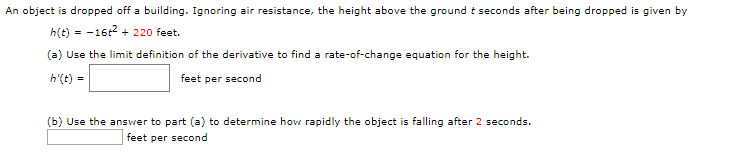An object is dropped off a building. Ignoring air resistance, the height above the ground t seconds after being droppedgiven byh(t)16 220 feet(a) Use the limit definition of the derivative to find a rate-of-change equation for the height.h'(t)feet per second(b) Use the answer to part (a) to determine how rapidly the object is falling after 2 seconds.feet per second

Questionhelp_outlineImage TranscriptioncloseAn object is dropped off a building. Ignoring air resistance, the height above the ground t seconds after being dropped given by h(t)16 220 feet (a) Use the limit definition of the derivative to find a rate-of-change equation for the height. h'(t) feet per second (b) Use the answer to part (a) to determine how rapidly the object is falling after 2 seconds. feet per second fullscreen
Step 1

Consider the given height function

Step 2

Subpart (a)

Now rate of change of the height

Step 3

Subpart (b)

So rate of change of height will be speed in term of t...

Want to see the full answer?

See Solution

Want to see this answer and more?

Our solutions are written by experts, many with advanced degrees, and available 24/7

See Solution
Tagged in

Derivative